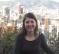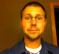## Code review. Newbie's first verilog module!Started by 6 years ago4 replieslatest reply 6 years ago290 views

Hi

This is one of the first verilog modules I wrote. It reads a 1-Wire iButton / Dallas keyfob code.

The theory of operation is that approximately every two seconds it samples the 1-Wire line and if a keyfob is present it transmits the "Read" command (0x33) and then reads back the 64-Bit code from the keyfob.

I wrote this about 18 Months ago as one of my first ever FPGA / #Verilog projects.

It does work, and works well, but I'm pretty sure it must be a suitable candidate for improving. So I invite you to give me your comments.

module iButton(
inout ibio,             // 1-Wire iButton/Dallas signal line.
input clk,                 // Master clock, currently 2.08 MHz
output [63:0]ibData,     // 64 Bit data read from iButton

reg ib_oe;
reg ibin, ds1, ds2;
reg [7:0] state ;
reg [31:0] count = 0;
reg [63:0] dataBitsIN = 64'd0;
reg [7:0] bitCount = 0;
reg [7:0] writeByte;
reg [7:0] lowCount;
reg [7:0] highCount;

parameter[7:0]
STATE_INITIAL_HOLD         = 8'h00,
STATE_START_BIT             = 8'h01,
STATE_START_HOLD         = 8'h02,
STATE_RELEASE_WAIT         = 8'h04,
STATE_BIT_WRITE_BEGIN    = 8'h05,
STATE_BIT_WRITE_SET         = 8'h06,
STATE_BIT_WRITE_WAIT    = 8'h07,
STATE_BIT_WRITE_RELEASE    = 8'h08,
STATE_BIT_HIGH_WAIT        = 8'h09,
STATE_BIT_WRITE_NEXT    = 8'h0a,

assign ibio = ib_oe  ?  1'b0 : 1'bZ; // High impedence, or driven low. Uses pull-up resistor for high.
assign ibData = (ibDataReady) ? dataBitsIN : 64'd0;

always @(posedge clk) begin
ds2 <= ds1;
ds1 <= ibio;
ibin <= ds2;
end

always @(posedge clk) begin
// Fail safe, resets the count and the state if we stall too long at any stage.
if(count > 32'h4F0000) begin
count <= 32'h0;
state <= STATE_INITIAL_HOLD;
end

case (state)
STATE_INITIAL_HOLD: begin // count to 3f7a00 (two seconds at 2.08 MHz) then move to next state;
if(count < 32'h3f7a00) begin
count <= count + 1;
end
else begin
count <= 0;
bitCount <= 0;
state <= STATE_START_BIT;
end
end
STATE_START_BIT: begin // transmit a >480 uS low pulse which wakes up and detects the iButton.
if (count < 32'd1100) begin
ib_oe <= 1'b1; // Output enable, drives low.
count <= count + 1;
end
else begin
ib_oe <= 1'b0; // release the io line
count <= 0; // reset the count for the next state.
state <= STATE_START_HOLD;
end
end
STATE_START_HOLD: begin // wait for 70 uS
if (count < 32'd146) begin
count <= count + 1;
end
else begin // move to the next state, sampling.
end
end
count <= 0;
if (ibin) begin // the iButton did not pull us low, so no iButton detected.
state <= STATE_INITIAL_HOLD;
end
else begin
state <= state + 1;
end
end
STATE_RELEASE_WAIT: begin // stay here until the line goes high again, and pause for a few uS.
if (ibin) begin
if(count > 100) begin
state <= state + 1;
writeByte <= 8'h33;
end
else begin
count <= count + 1;
end
end
end
STATE_BIT_WRITE_BEGIN: begin // write READ_ROM Command (0x33);
if(bitCount < 8) begin // preload the low and high counts. Calculated at 2.08 MHZ
if (writeByte) begin
lowCount <= 8'd13;
highCount <= 8'd132;
state <= state +1;
end
else begin
lowCount <= 8'd125;
highCount <= 8'd10;
state <= state +1;
end
end
else begin
count <= 0;
end

end
STATE_BIT_WRITE_SET: begin // write the low mark
ib_oe <= 1'b1;
state <= state +1;
end
STATE_BIT_WRITE_WAIT: begin // wait for the lowCount
if (lowCount == 0) begin
state <= state + 1'b1;
end
else begin
lowCount <= lowCount - 1'b1;
end
end
STATE_BIT_WRITE_RELEASE: begin // set the pin high
ib_oe <= 0; // switch off the drive, allow to float high.
state <= state + 1;
end
STATE_BIT_HIGH_WAIT: begin // wait for the high count
if (highCount == 0) begin
state <= state + 1;
end
else begin
highCount <= highCount - 1'b1;
end
end
bitCount <= bitCount + 1;
writeByte <= {1'b0, writeByte[7:1]}; // rotate writeByte by 1 bit right.
state <= STATE_BIT_WRITE_BEGIN; // loop for remaining bits
end
STATE_BIT_READ_BEGIN: begin // We have finished writing the command to read the rom. Now lets get set up to read back 64 Bits.
bitCount <= 0;
dataBitsIN <= 64'd0;
state <= state + 1;
end
STATE_BIT_READ_ASSERT: begin // write a 0 on the line
if (bitCount < 40) begin
dataBitsIN <= {1'b0, dataBitsIN[63:1]};
ib_oe <= 1'b1; // drive line low
count <= 0;
state <= state + 1;
end
else begin
count <= 0;
state <= 0; // start again, waiting for key presence.
end
end
STATE_BIT_READ_HOLD1: begin // wait for 6 uS
if (count < 12) begin
count <= count + 1;
end
else begin // stop driving line
ib_oe <= 1'b0; // release the line
state <= state + 1;
count <= 0;
end
end
STATE_BIT_READ_HOLD2: begin // Wait for 9 uS
if (count > 18) begin
state <= state + 1;
end
end
bitCount <= bitCount + 1;
if (ibin) begin // If we read back a '1' bit.
dataBitsIN <= {1'b1, dataBitsIN[62:0]}; // no need to do anything for a '0' bit as MSb is already '0'.
end
end
default: begin
state <= 8'h00;
count <= 0;
end
endcase
end

endmodule
[ - ]Small suggestions on code writing.

If you may change your clk frequency at some point, I recommend that you use external parameter defines to set the count values. This will allow you to reuse the module and instantiate in other clk domains without having to reedit the module every time ;)

And also I do prefer having a global reset, but it depends on what are your preferences.

When you go to the next state, I prefer always have the name of the next state rather than having state + 1. It makes the code more readable.

[ - ]I won't comment on the functionality but here are some suggestions for making your module more reusable.

module iButton(

Never hard code constants in a program. Always use a variable in case someone tries to reuse your module in a design that already has a module of the same name.

ie:

iButton_defines.v

ifndef  BASENAME

define  BASENAME  iButton

endif

iButton.v

module BASENAME

Do it this way and the user can easily override your name.

Resets:

Always include and active low asynchronous reset and an active high synchronous reset for each flip flop. The users can tie them off if not needed.

Never do this:

reg [31:0] count = 0;
reg [63:0] dataBitsIN = 64'd0;
reg [7:0] bitCount = 0;

It doesn't synthesize, use a reset.

Never do this inside of a module

assign ibio = ib_oe  ?  1'b0 : 1'bZ; // High impedence, or driven low. Uses pull-up resistor for high.


Create a pad model and use that. Fpga tools can handle this but asic tools cannot

The same is true for synchronous rams. Create a generic model for your source that can be replaced by a hard macro for an asic design.

No magic numbers!

I always cringe when I see stuff like this:

         if (count < 32'd146) begin

Define a parameter or a define and use that instead.

John Eaton

[ - ]This will synthesize with most FPGA tools

reg [31:0] count = 0;
reg [63:0] dataBitsIN = 64'd0;
reg [7:0] bitCount = 0;
`

And is often recommend as the initialization process instead of a global reset in an FPGA.

I really do not like the ifdef module name nor do I see much benefit to it.

I agree with the no magic numbers, include localparam at the beginning of the module with the definitions.

[ - ]